# Making games with Falling Sand part 1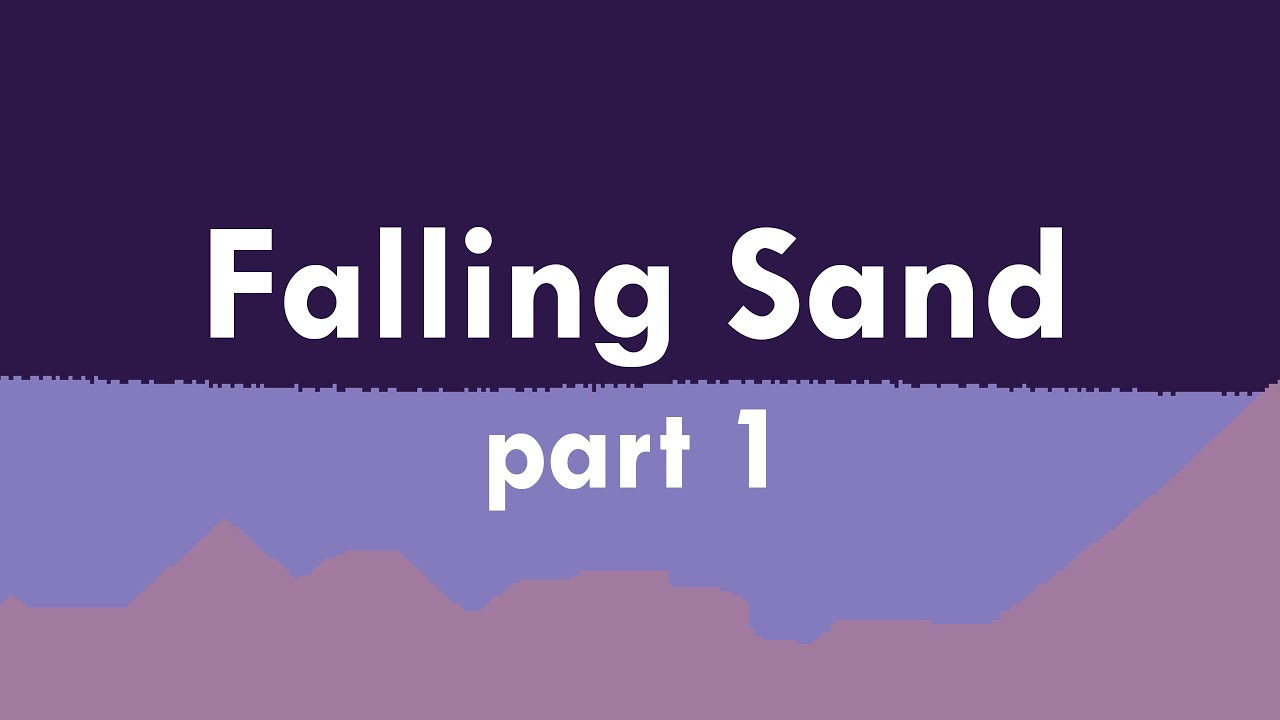Simulating falling sand is no different from any other cellular automata. We have a big gird of cells and a set of rules that get applied to each cell every frame. You've probably heard of the Game of Life. In that cellular automata, the rules are based on the number of neighbors surrounding a cell. For falling sand, we mostly look at if there is empty space below a cell for it to move into. This creates the look of particles cascading down.

One of the more interesting aspects of cellular automata is how complex behavior can emerge from such simple rules. That sounds like an interesting project could be created from only a few lines of code! Let's look at how you could go about making one of these in the Java version of Processing. You can download Processing here if you want to follow along.

## Java version #

We'll start with the standard setup and draw functions. Before we fill these in, we should establish what our particles will be. For simplicity, color will determine the particle types, so let's define some colors at the top.

sketch.pde

```color _EMPTY = color(0);
color _SAND  = color(255, 150, 50);

void setup() {
}

void draw() {
}
```

In setup we need to set the size and background. I like to use a size of 800x800 pixels, and we need to set the background to the empty particle color. We should also uncap the framerate from 60 to allow this to run faster if it can.

```void setup() {
size(800, 800);
background(_EMPTY);
frameRate(1000);
}
```

Each time the draw function is called we will step our simulation. Because we are only using color, let's use the pixels array as the storage for our cells. Each frame we need to call loadPixels to copy the displayed frame into the pixels array. This first frame will be all _EMPTY because we called background.

```void draw() {
```

After we load the pixels, we can iterate over them and check if they match a particle type that we know about.

```  for (int x = 0; x < width;  x++)
for (int y = 0; y < height; y++) {
color cell = get(x, y);

if (cell == _SAND) {
```

We want sand to form dune like shapes, so let's encode a pyramid into its rules. We need to check directly below, down to the left, and down to the right.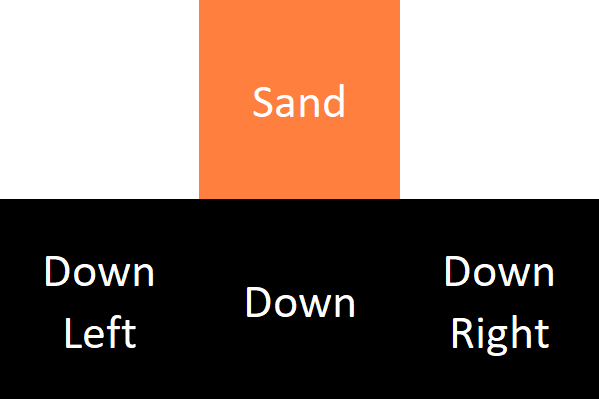By default, it should move down if possible, but if that cell is occupied, we'll check the other two directions.

```		boolean down  = isEmpty(x,     y + 1);
boolean left  = isEmpty(x - 1, y + 1);
boolean right = isEmpty(x + 1, y + 1);

if(down)  setCell(x,     y + 1, _SAND);
else if(left)  setCell(x - 1, y + 1, _SAND);
else if(right) setCell(x + 1, y + 1, _SAND);

if (down || left || right) {
setCell(x, y, _EMPTY);
}
}
```

This looks ok, but the order of if statements matters. Because we put the left check before right, if both spaces are open, the particle will always move left. This creates an artificial look, but we can easily fix it by adding a little randomness to shuffle the direction if both cells are empty.

```	if (left && right) {
boolean rand = random(1) > .5;
left  = rand;
right = !rand;
}
```

Finally, we can close the for loops and call updatePixels to copy our frame to the display.

```	}

updatePixels();
}
```

To draw particles on the screen with the mouse, we can add these lines after loadPixels.

```	if (mousePressed) {
for (int x = 0; x < 1; x++)
for (int y = 0; y < 1; y++) {
setCell(mouseX + x, mouseY + y, _SAND);
}
}
```

You can create other particle types by adding more rules in a similar way. For example, water and a stationary barrier type are the most straight forward and where I would start. Water is like sand, but we check directly to the left and right instead of diagonal, which gives the effect of liquid flow. Barriers have no movement properties but fill space so the other types can't move through them.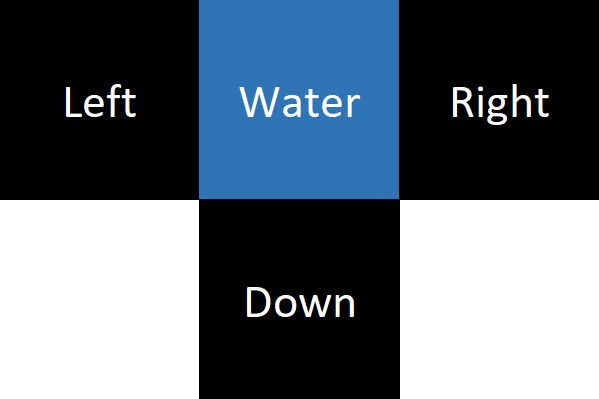### Common issues

I saved the helper functions for last to highlight two common issues. The biggest gotcha with these types of simulations is that the order of iteration effects the behavior dramatically, and if we aren't careful, we could end up updating particles multiple times or even lose them entirely.

sketch.pde

```boolean inBounds(int x, int y) {
return x >= 0    && y >= 0
&& x < width && y < height;
}

boolean isEmpty(int x, int y) {
return inBounds(x, y) && pixels[x + y * width] == _EMPTY;
}

void setCell(int x, int y, color cell) {
pixels[x + y * width] = cell;
}
```

We have four options for how we order the iteration. No matter which we pick, there will always be slight issues. Let's see why.

If we iterate top to bottom, we end up updating the same particle multiple times. This results in a teleportation effect where a particle will only take a single frame to reach the floor. We could change the iteration to bottom to top, but then if we want particles that moves up, we run into the same issue. This highlights the fact that no matter which direction we pick, there will always be issues. Clearly, the solution must have more to it than just finding a magic ordering...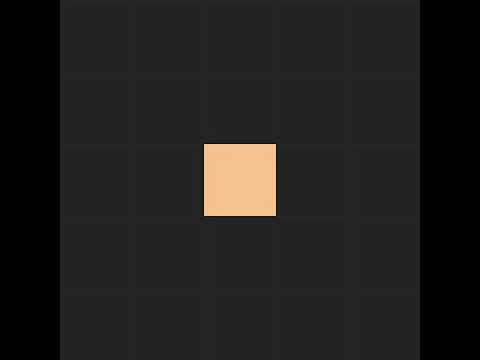The band aid solution in this Processing version comes from the subtle details of how the pixel array works. In the helper functions we read and write to the pixels array, but in draw we use the get function. Even though the docs don't say this, get must read from the displayed pixels array, which we don't update until we call updatePixels. This allows us to dodge the issue of updating particles multiple times because nothing updates until the end of the frame from get's perspective.

The second problem we can only mitigate. In isEmpty we don't use get because we want information about the current frame as it updates. If we used get, two particles could both think a cell is empty, move in, and one would be lost. Even though we've somewhat fixed that issue, the order of iteration still matters. Now the order on the X axis determines who moves in first, so we still have inconsistent behavior.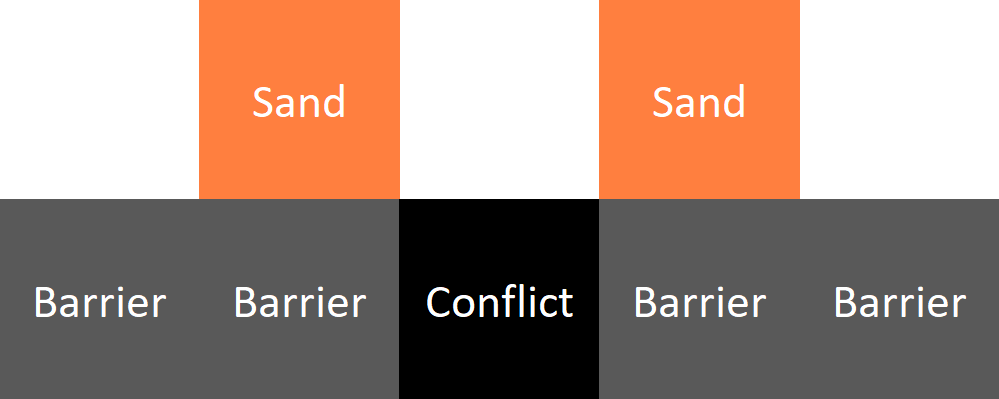Now that we have an idea of how the basics work, and common pitfalls, let's jump over to C++ and see how these can be solved.

## C++ Version #

I plan on expanding this over the next few videos, so I am going to try and make it more of a general sand framework. To start, we don't want to edit the pixels directly because we want more properties than just color. Let's make a CellType enum and store it in a Cell struct along with a Color. In the first version, sand and water both needed to check if the space directly below was free. This hints that the ways that the particles move doesn't have to reflect their type. So, let's keep two enums in each Cell, one for the movement properties and another the type. If we use a bit string for the properties, they can be combined to create more complex patterns.

Cell.h

```enum CellProperties {
NONE           = 0b00000000,
MOVE_DOWN      = 0b00000001,
MOVE_DOWN_SIDE = 0b00000010,
MOVE_SIDE      = 0b00000100
};

inline CellProperties operator|(CellProperties a, CellProperties b) {
return CellProperties((int)a | (int)b);
}

inline auto operator&(CellProperties a, CellProperties b) {
return (int)a & (int)b;
}

enum CellType {
EMPTY,
SAND,
WATER,
ROCK
};

struct Cell {
CellType       Type  = CellType::EMPTY;
CellProperties Props = CellProperties::NONE;

Color Color; // rgba
};
```

Now that we have defined our Cell, we need a way to store them. Let's make a new class called SandWorld and store an array of Cells in it. In the constructor we can specify a width, height, and scale in pixels. This will allow us to easily change the size of the cells.

SandWorld.h

```class SandWorld {
public:
const size_t m_width  = 0;
const size_t m_height = 0;
const double m_scale = 1;
private:
Cell* m_cells = nullptr;

public:
SandWorld(size_t width, size_t height, double scale)
: m_width  (width  / scale)
, m_height (height / scale)
, m_scale  (scale)
{
m_cells = new Cell[m_width * m_height];
}

~SandWorld() {
delete[] m_cells;
}
```

We'll need some functions for getting the cells out of the world, let's add two: one that takes x and y coordinates, and another that takes a flat index.

```	const Cell& GetCell(size_t index) {
return m_cells[index];
}

const Cell& GetCell(size_t x, size_t y) {
return GetCell(GetIndex(x, y));
}

size_t GetIndex(size_t x, size_t y) {
return x + y * m_width;
}
```

And to get us back to where we were, let's add the same helper functions from before.

```	bool InBounds(size_t x, size_t y) {
return x < m_width
&& y < m_height;
}

bool IsEmpty(size_t x, size_t y) {
return InBounds(x, y)
&& GetCell(x, y).Type == CellType::EMPTY;
}

void SetCell(size_t x, size_t y, const Cell& cell) {
m_cells[GetIndex(x, y)] = cell;
}
```

Processing gave us two arrays to work with, but before we just add another one and call it a day, let's think about a way to actually solve the issues that arise from the iteration ordering. The main problem is that moves are executed as they come, but really, we should gather all the possible moves, then execute them at the end to give each one a fair chance.

Let's add a vector to the SandWorld, and make a new function called MoveCell that adds a move to the list.

```	std::vector<std::pair<size_t, size_t>> m_changes; // destination, source

void MoveCell(size_t x, size_t y, size_t xto, size_t yto)
{
m_changes.emplace_back(
GetIndex(xto, yto),
GetIndex(x,   y)
);
}
```

After we finish iterating over our cells, we can apply the changes. First, we need to remove any changes that were filled between frames by the SetCell function.

```	void CommitCells() {
// remove moves that have their destinations filled

for (size_t i = 0; i < m_changes.size(); i++) {
if (m_cells[m_changes[i].first].Type != CellType::EMPTY) {
m_changes[i] = m_changes.back(); m_changes.pop_back();
i--;
}
}
```

Then we need to sort the list of moves by their destination. This is an unfortunate slowdown but allows us to add and choose moves quicker. We could use a multimap, but the slowdown from accessing the linked lists outweighs the sort.

```		// sort by destination

std::sort(m_changes.begin(), m_changes.end(),
[](auto& a, auto& b) { return a.first < b.first; }
);
```

Then we can iterate over the sorted moves. Each time the destination changes, we'll pick a random source to move from. This allows each particle to get a fair chance at moving into to a cell. Finally, we'll clear the list.

```		// pick random source for each destination

size_t iprev = 0;

m_changes.emplace_back(-1, -1); // to catch final move

for (size_t i = 0; i < m_changes.size() - 1; i++) {
if (m_changes[i + 1].first != m_changes[i].first) {
size_t rand = iprev + rand_int(i - iprev);

size_t dst = m_changes[rand].first;
size_t src = m_changes[rand].second;

m_cells[dst] = m_cells[src];
m_cells[src] = Cell();

iprev = i + 1;
}
}

m_changes.clear();
}
};
```

That's it for the core of our little framework, let's see how we can use it to make what we had in the Processing version. I am going to skip over the rendering details because C++ makes that really annoying. I suggest checking out SDL if you don't already have your own solution.

In an Update function, we'll iterate over the cells in a similar way as before, but now that we have a bit string of movement properties, we can check each one until its respective MoveX function returns true.

Main.cpp

```SandWorld m_world = SandWorld(1920, 1080, 2);

void Update() {
// Update cells

for (size_t x = 0; x < m_world.m_width;  x++)
for (size_t y = 0; y < m_world.m_height; y++) {
const Cell& cell = m_world.GetCell(x, y);

if (cell.Props & CellProperties::MOVE_DOWN      && MoveDown    (x, y, cell)) {}
else if (cell.Props & CellProperties::MOVE_DOWN_SIDE && MoveDownSide(x, y, cell)) {}
else if (cell.Props & CellProperties::MOVE_SIDE      && MoveSide    (x, y, cell)) {}
}

m_world.CommitCells();

// Update the sand texture
// Draw the sand to the screen
}
```

We can make a function for each movement property that will return true if it finds a valid move.

```bool MoveDown(size_t x, size_t y, const Cell& cell)
{
bool down = m_world.IsEmpty(x, y - 1);
if (down) {
m_world.MoveCell(x, y, x, y - 1);
}

return down;
}

bool MoveDownSide(size_t x, size_t y, const Cell& cell)
{
bool downLeft  = m_world.IsEmpty(x - 1, y - 1);
bool downRight = m_world.IsEmpty(x + 1, y - 1);

if (downLeft && downRight) {
downLeft  = rand_float() > 0;
downRight = !downLeft;
}

if (downLeft)  m_world.MoveCell(x, y, x - 1, y - 1);
else if (downRight) m_world.MoveCell(x, y, x + 1, y - 1);

return downLeft || downRight;
}
```

To draw particles with the mouse, we can create some defaults in an Init function...

```Cell _EMPTY, _SAND, _WATER, _ROCK;

void Initialize() {
_EMPTY = {
CellType::EMPTY,
CellProperties::NONE,
Color::From255(0, 0, 0, 0) // 0 alpha allows for a background
};

_SAND = {
CellType::SAND,
CellProperties::MOVE_DOWN | CellProperties::MOVE_DOWN_SIDE,
Color::From255(235, 200, 175)
};

_WATER = {
CellType::WATER,
CellProperties::MOVE_DOWN | CellProperties::MOVE_SIDE,
Color::From255(175, 200, 235)
};

_ROCK = {
CellType::ROCK,
CellProperties::NONE,
Color::From255(200, 200, 200)
};

// Init a texture for sand...
}
```

Then at the top of our update function, we'll add these lines.

```void Update() {
if (Mouse::ButtonDown(LMOUSE)) {
vector2 pos = Mouse::ScreenPos() / m_world.m_scale;
pos.y = m_world.m_height - pos.y;

Cell placeMe = _EMPTY;

if (Keyboard::KeyDown(S)) placeMe = _SAND;
else if (Keyboard::KeyDown(W)) placeMe = _WATER;
else if (Keyboard::KeyDown(R)) placeMe = _ROCK;

for (size_t x = pos.x; x < pos.x + 20; x++)
for (size_t y = pos.y; y < pos.y + 20; y++)
{
if (!m_world.InBounds(x, y))
continue;

m_world.SetCell(x, y, placeMe);
}
}

// Update cells
// Copy sand colors to a texture
// Draw the texture on the screen
}
```

The last thing I want to cover is about making games inside of these simulations. Let's think about the most basic feature we need, then in future posts I'll expand on this.

Having a player that moves around seems like a good place to start, so let's think about how that might work. We'll need some notion of a group of particles that can move together. Let's make a Tile struct that holds a list of positions for its particles, and a position for the group itself. For now, we'll just use the _ROCK particle for everything in the tile.

Tile.h

```struct Tile {
std::vector<std::pair<int, int>> Positions;
int X = 0;
int Y = 0;
};
```

Before we update the world, we need to paste the tile particles in, so let's put these lines in the Update function before the main loops. After we've called CommitCells and updated the texture, we can remove the tiles by setting their cells to _EMPTY.

Main.cpp

```std::vector<Tile> m_tiles;

void Update() {
// Draw cells with mouse

for (Tile& tile : m_tiles) {
for (auto [x, y] : tile.Positions) {
x += tile.X;
y += tile.Y;

if (m_world.InBounds(x, y)) {
// what happens if the cell is already full?
m_world.SetCell(x, y, _ROCK);
}
}
}

// Update cells
// Copy sand colors to a texture
// Draw the texture on the screen

for (Tile& tile : m_tiles) {
for (auto [x, y] : tile.Positions) {
x += tile.X;
y += tile.Y;

if (m_world.InBounds(x, y)) {
// what happens if the cell is no longer part of the tile?
m_world.SetCell(x, y, _EMPTY);
}
}
}
}
```

Finally, we can make a little ship and add some basic movement. In the Init function, we'll make a Tile and add it to the list.

```void Initialize() {
Tile ship = {
{
{2,6},
{1,5}, {2,5},
{0,4}, {1,4}, {2,4}, {3,4},
{0,3}, {1,3}, {2,3}, {3,3},
{0,2},               {3,2},
{0,1},               {3,1},
{0,0},               {3,0},
},
200, 200
};

m_tiles.push_back(ship);

// Create default cells
// Init a texture for sand...
}
```

In the Update function, before the world updates, we can add these lines to move our ship. It's important to move the tiles before pasting them in so they can be removed correctly at the end.

```void Update() {
// Draw cells with mouse

if (Keyboard::KeyDown(LEFT))  m_tiles.X -= 1;
if (Keyboard::KeyDown(RIGHT)) m_tiles.X += 1;
if (Keyboard::KeyDown(UP))    m_tiles.Y += 1;
if (Keyboard::KeyDown(DOWN))  m_tiles.Y -= 1;

// Paste tiles
// Update cells
// Copy sand colors to a texture
// Draw the texture on the screen
// Remove tiles
}
```

And that about covers it, we're left with a decently quick simulation. On my computer this runs between 0.005 - .015 seconds per frame. Which is around 200 - 60 fps. Currently we can only use 1 thread, so if your computer has 4 cores we are only using 1/4th or more likely only 1/8th of its power :(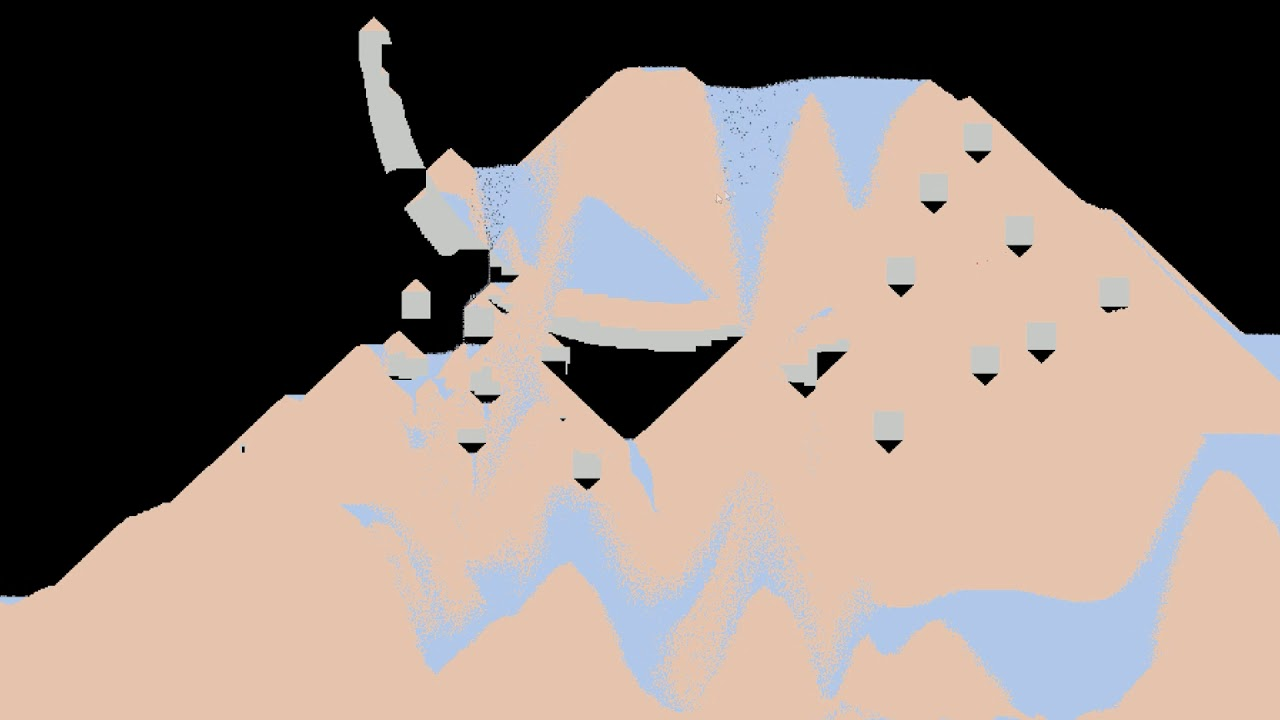In the next post, I am going to cover how we could make the world bigger, and how multi-threading the simulation works. Then we'll make a game with it, so stay tuned if this seems interesting!